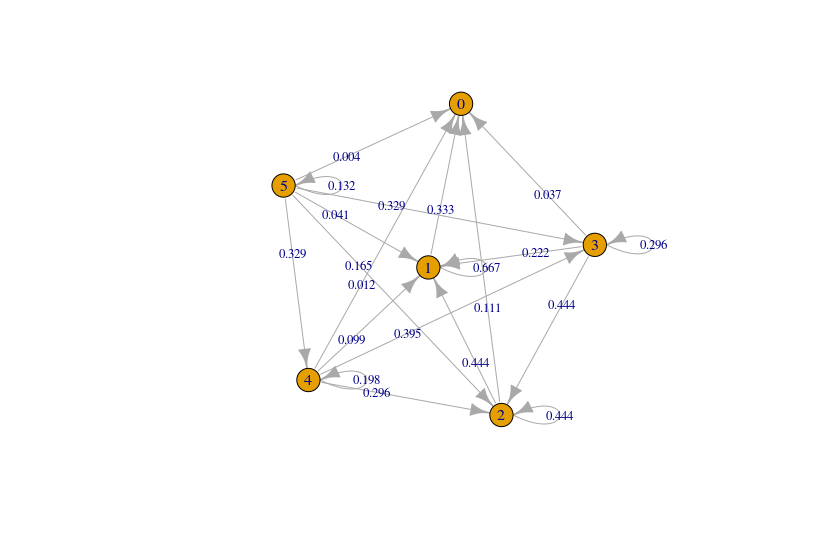Want to share your content on R-bloggers? click here if you have a blog, or here if you don't.“What is the probability of ending a round with zero points in Dr. Burnörium’s Psycho Dice Russian Roulette Game?” That question came up during a Psycho Dice gaming session, when we noticed that turns ending with zero points occur about a quarter of the time. I decided to check if the probability of getting zero points in theory is consistent with our observations in practice.

### Goal

The goal of the game is to get the highest score after six rounds of rolling dice – or at least to avoid getting the lowest score because the loser has to eat a Chocolate Bullet. Although some are harmless and sweet, the majority contain capsaicin and are painful to eat.

### Scoring Rules

Points in the game are won by throwing six-sided dice, each containing 4 numbers and 2 bullets.

You start your roll each round with five dice. This is how a roll is scored:

1. If your roll shows numbers only, you get points. (The sum of the numbers and additional points if multiple dice landed on the same number.)
2. If at least one die shows a bullet, the roll is worth zero points. Each die showing a bullet is discarded in the next roll, so if two of your five dice show a bullet for example, you will throw three dice in your next roll.

## Possible Paths to Zero Points

For each roll, I generated the probabilities of rolling between 0 and N bullets, where N is the number of dice in a roll. Then I used the igraph package to create a graph of possible paths from rolling 5 dice to 0 dice with the `graph_from_data_frame()` function.The nodes in the graph represent the number of dice in the current roll; the edges are paths to the number of dice in the next roll. Each edge is associated with the probability of taking that path. For example, if you throw five dice and get one bullet, you’ll move from node 5 to node 4. (There is a 32.9% chance of that happening.)

Each node also has a loop because you’ll throw the same number of dice again if you don’t get a bullet dice. The probability of scoring points is 13.2% when throwing five dice, but it increases to 66.7% by the time you’re left with one die.

A loop occurs when you don’t get any bullets in a roll, so I decided to use a simple paths analysis to make sure each node is included in the path only once. Running the `igraph::all_simple_paths()` function returned 16 paths to zero dice without scoring a single point. All throws are independent because the number of bullets you get in each roll does not depend on previous rolls. Therefore, we multiply the probabilities on each path to get the probability of taking that path and sum them all up to find the probability of ending a round with zero points.

Path ID Path to 0 points Probability
1 5 -> 1 -> 0 0.0136530
2 5 -> 2 -> 1 -> 0 0.0243956
3 5 -> 2 -> 0 0.0183150
4 5 -> 3 -> 1 -> 0 0.0243217
5 5 -> 3 -> 2 -> 1 -> 0 0.0215976
6 5 -> 3 -> 2 -> 0 0.0162144
7 5 -> 3 -> 0 0.0121730
8 5 -> 4 -> 1 -> 0 0.0108461
9 5 -> 4 -> 2 -> 1 -> 0 0.0143984
10 5 -> 4 -> 2 -> 0 0.0108096
11 5 -> 4 -> 3 -> 1 -> 0 0.0096071
12 5 -> 4 -> 3 -> 2 -> 1 -> 0 0.0085311
13 5 -> 4 -> 3 -> 2 -> 0 0.0064047
14 5 -> 4 -> 3 -> 0 0.0048083
15 5 -> 4 -> 0 0.0039480
16 5 -> 0 0.0040000
TOTAL   0.2040236

Overall, assuming that all outcomes of rolling the dice are equally likely to occur, there is a 20.40% chance of scoring zero points in Dr. Burnörium’s Psycho Dice Russian Roulette Game, which is close to what we observed during the game. We didn’t keep the previous score sheets, so I’ll have to give the game another go one day to verify the result in practice.